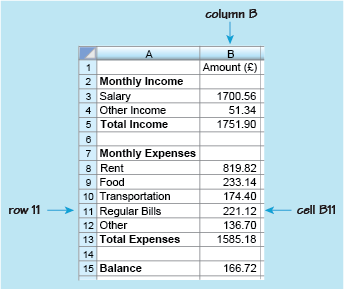Succeed with maths: part 2

Start this free course now. Just create an account and sign in. Enrol and complete the course for a free statement of participation or digital badge if available.

Last week, the course looked briefly at how to construct a word formula but concentrated on how to use formulas you were given successfully. This week moves onto building your own formulas, starting with a practical application using spreadsheets. As well as having relevance to future university level studies, if you need to work with data that you or somebody else has collected, being able to work with spreadsheets may well come in useful at home or at work, now and in the future. It is also a useful way to learn more about constructing your own formulas.

Before getting going though, it is worth pausing to think about what a spreadsheet is, how they may be useful and the way they are set up.

At the basic level, spreadsheets can be used to display data, such as fuel prices over a number of years, in tables. Once, the data are in a spreadsheet then the real strengths of spreadsheets can be used. You can perform calculations on the data to work out other information from the data and even use a spreadsheet to create charts for you to display the data or results. Spreadsheets come into their own when you have a mountain of data and calculations to perform on it.

Figure 1 shows part of a spreadsheet that has been constructed to record monthly income and outgoings, or expenditure. It is similar to a bank balance sheet that you might draw up by hand to check your monthly income and expenses, the totals, and the overall balance. However, the spreadsheet has been created on a computer and has formulas inserted into it to enable automatic calculations without using a calculator.Figure _unit5.1.1 Figure 1 A spreadsheet used for a monthly budget

As with any other spreadsheet, this example is made up of rows and columns containing boxes. These boxes are called ‘cells’. The columns are identified by letters and the rows by numbers. This enables you to identify each cell in the spreadsheet. For example in this spreadsheet, the number 221.12 is in column B, row 11, so is in cell B11.

Cells can thus be found, from their reference, by looking down the column and across the row. So cell A3 can be found by looking down column A and across row 3. This cell contains the word ‘Salary’. Notice that cells can contain either text or numbers.

Use this knowledge when completing your first activity of the week.

## Activity _unit5.1.1 Activity 1 Spreadsheet cells

Timing: Allow approximately 5 minutes

• a.What word or number is contained in the following cells?
• i.A5
• ii.A12
• iii.B15
• iv.B1

• a.

• i.The cell that is in both column A and row 5 contains the words ‘Total Income’.
• ii.Down column A and across row 12, cell A12 contains the word ‘Other’.
• iii.The cell that is in column B, row 15 contains the number 166.72.
• iv.The cell that is in column B, row 1 contains the heading ‘Amount (£)’.
• b.What is the reference for the cells that contain the following?
• i.The number 1585.18.
• ii.The word ‘Food’.

• b.

• i.1585.18 is in column B and row 13, so its reference is B13.
• ii.‘Food’ is in column A and row 9, so its reference is A9.

So, remember when you are referring to a particular cell, you always state the column letter, followed by the row number.

Looking again at Figure 1 you can see what other information it shows. For example, if you look at row 3, this shows that the monthly salary is £1700.56. Although cell B3 only contains the number 1700.56, you know that this is measured in pounds from the heading in cell B1 ‘Amount (£)’. Overall, the spreadsheet shows the items that make up the monthly income and where money has been spent over the month. If you were keeping these records by hand, you would then need to calculate the total income, the total expenses and the balance.

For example, to find the total income for the month, you would need to add the salary of £1700.56 to the other income of £51.34. This gives the total income of £1751.90. In other words, to calculate the value in cell B5, you need to add the values in cells B3 and B4. This can be written as the following formula: